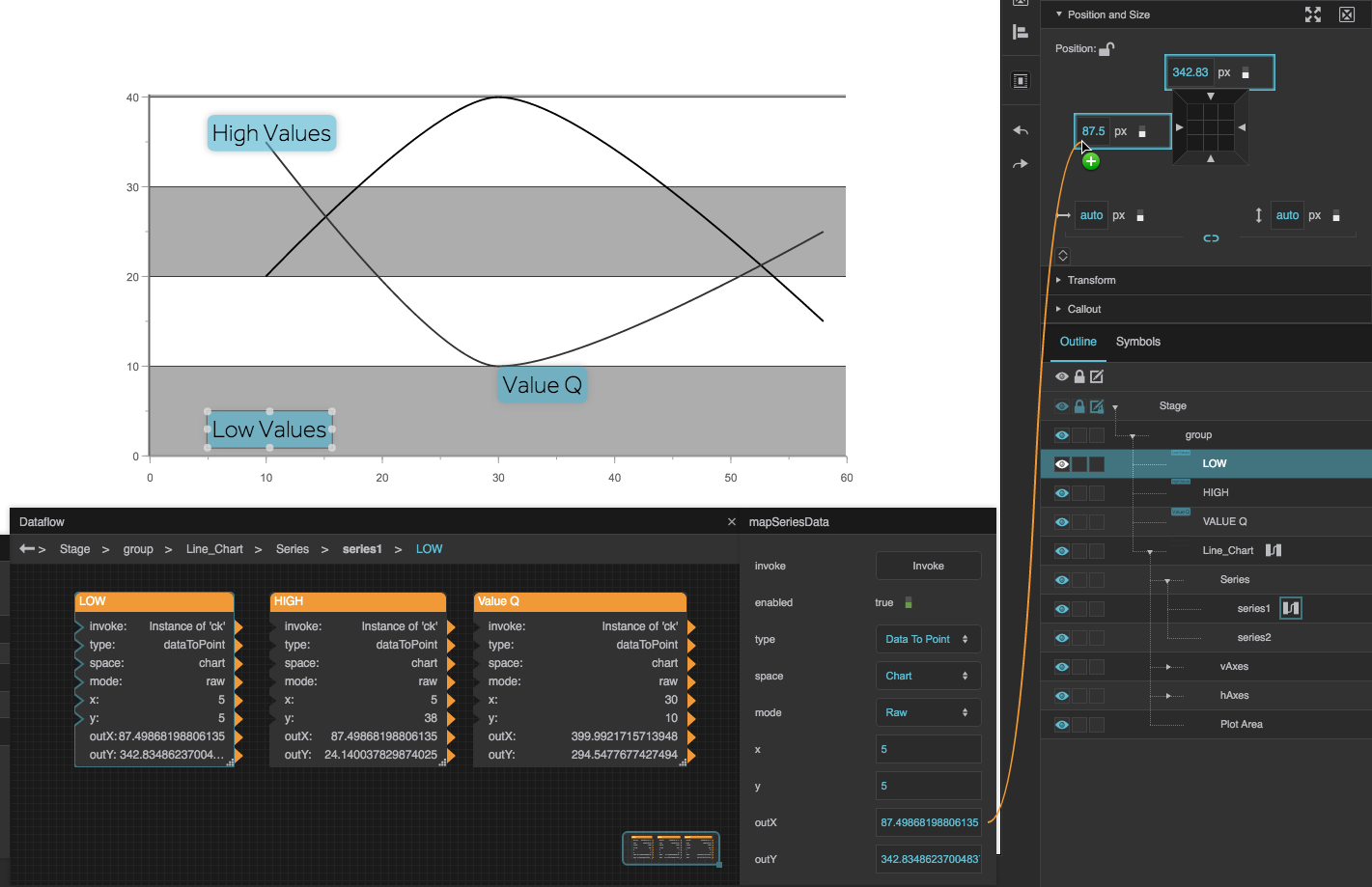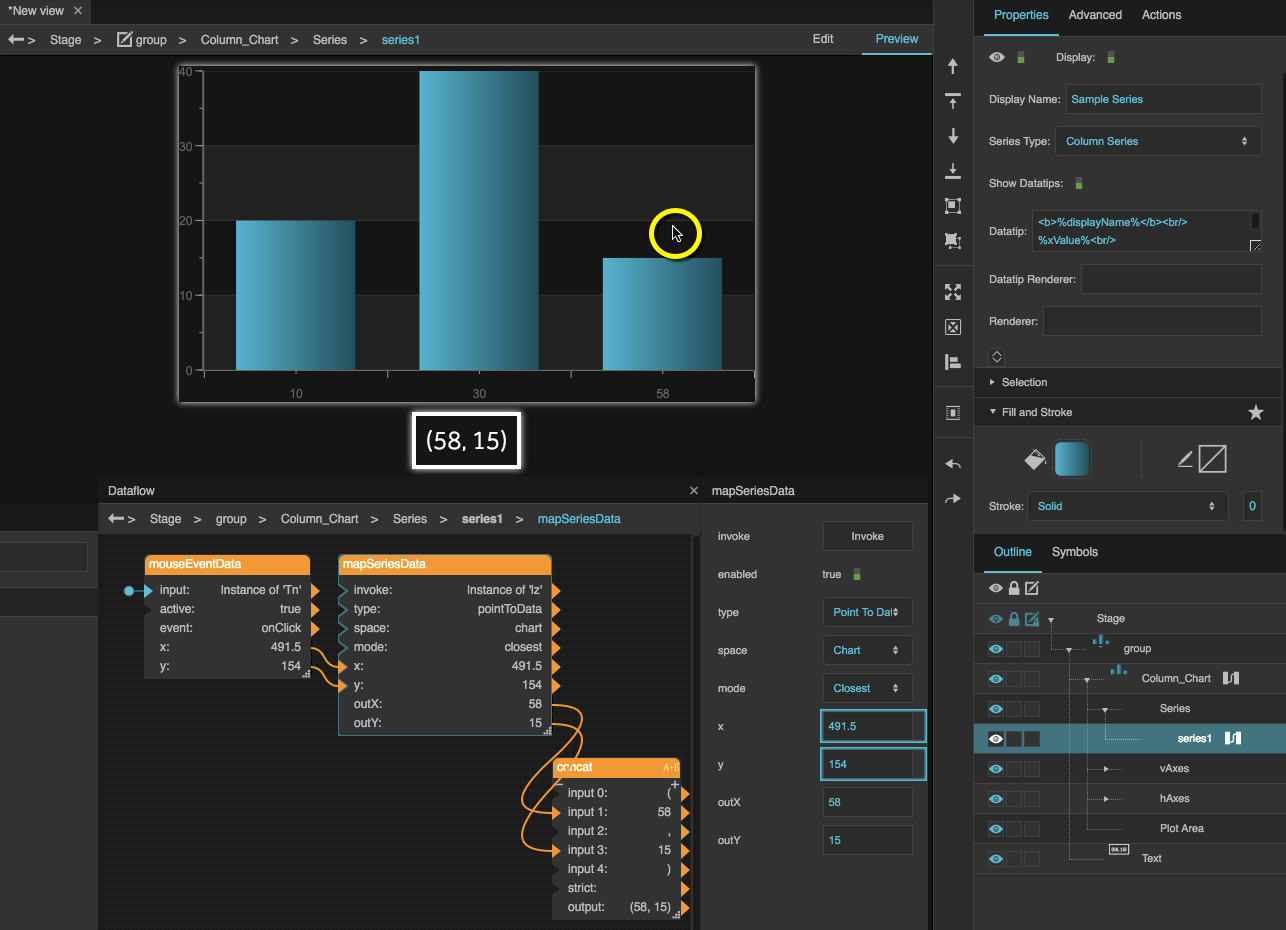# Map Series Data

 Block Group: Logic Icon: no icon

Converts between X and Y coordinates that are relative to a DGLux5 space and X and Y coordinates that are relative to a chart series.

In the context of this block:

• "Point coordinates" are relative to a DGLux5 space, such as a component. The X point coordinate is the number of pixels from the left edge of the space, and the Y point coordinate is a number of pixels from the top edge.
• "Data coordinates" are relative to a chart series. They are locations on the series' X and Y axes.

In order for this block to work, the parent of this block must be the series whose data is part of the conversion.

For information on using dataflow blocks, see Dataflow.

## Input/Output Properties

These properties can take input and give output.

• invoke (trigger)
• enabled (boolean)
• type (enum)
• space (enum)
• mode (enum)
• x (number)
• y (number)

invoke causes the values to be converted. Only works if enabled is set to TRUE.

enabled specifies whether the values will be converted.

• TRUE: The values are converted when invoke is triggered.
• FALSE: The values are not converted.

type specifies the direction of the conversion that this block performs.

• PointToData: The input values are point coordinates. The output values are data coordinates.
• DataToPoint: The input values are data coordinates. The output values are point coordinates.

x and y are the coordinates before conversion. If this is a PointToData conversion, they are point coordinates. If this is a DataToPoint conversion, they are data coordinates.

## Output Properties

These properties can give output. They cannot take input.

• outX (number)
• outY (number)

outX and outY are the coordinates after conversion. If this is a PointToData conversion, they are data coordinates. If this is a DataToPoint conversion, they are point coordinates.

## Model

These are some basic uses of the block.

Dataflow Model DescriptionThe top and left edges of the text components will always appear at the same chart coordinates, even if the group is resized.

In this example:

• The text components are grouped together with the chart.
• DataToPoint is selected for type.
• Chart is selected for space.
• Raw is selected for mode.
• The output point coordinates are bound to each shape's position properties.When the user clicks the chart, the data coordinates for the nearest data point in the series are displayed.

In this example:

• The onClick Advanced property for the chart component is the input for the Mouse Event block.
• The x and y properties from the Mouse Event block are the inputs for the Map Series Data block
• PointToData is selected for type.
• Chart is selected for space.
• Closest is selected for mode.
• The outputX and outputY properties from the Map Series Data block are concatenated into a string that is bound to the Text property of a text component.